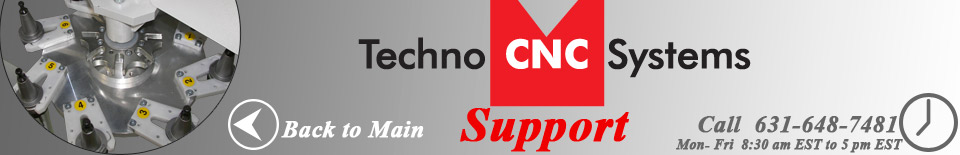Scale Factors

The scale factors are numbers that represent the smallest increment that can be distinguished per unit length. They're used to inform the software of how much linear distance corresponds to the distance between encoder markers.

Scale factors are printed on the front leg of all new routers.

## Contents

### Scale Factor Setup

Scale factors can be changed by going to Setup > System, and changing the M1 through M4 scales. A negative sign indicates direction.

• Click on Setup > System
• Find the "Mx Scale" section.
• Change the Scale Factor(s) to the correct value(s) for the machine. See the Scale Factor label on the gantry or front leg for details. Otherwise, see below for a guess.
• Click "OK" to save change

If the scale factors are unavailable, try the following values, depending on your machine:

### LC

• All axes: -20320

• M1: 20320
• M2: -20320
• M3: -20320

• M1: -12192
• M2: -7620
• M3: -20320

### RG

• M1: -10160 (most) or -12192 (59"x50")
• M2: -7620 (newer) or -5080 (older)
• M3: -20320

• M1: 10160
• M2: 5080
• M3: -20320

If none of these values work, please check your measurement device. Try measuring over a larger, or shorter area for verification.

### Manual Scale Factor Generation

If you are still having problems, perform the following operation:

• Using calipers, measure exactly how far the machine moves when you use jog step to move the machine 1 inch.
• Take the current scale factor value, and divide it by the result you just got. (i.e. 20320 / .9")

The resulting number is the correct scale factor. Repeat this test until you achieve the desired accuracy. The number will likely be a round number.

### Mathematical Scale Factor Generation

On some machines, unusual pulleys have been used. This results in scale factors unlike the typical ones listed above. To obtain an exact scale factor for these, some simple math is necessary.

• Count the number of teeth on the motor pulley and ball screw pulley
• The number of pulses per revolution on a standard motor is 4000.
• The typical screw pitches are 5,10 and 20 mm. Spin the screw once while measuring the distance traveled and ascertain which pitch screw you have.

Using these numbers, you can calculate what the appropriate scale factor is.

Scale Factor for 1 inch = ((1/(screw pitch /25.4))*4000)*(ball screw pulley teeth /motor pulley teeth) Scale Factor for 1 millimeter = ((1/(screw pitch))*4000)*(ball screw pulley teeth /motor pulley teeth)

So a scale factor for 1" units:

• On a 20mm screw
• With 4000 pulses per rev of the motor
• 20 teeth on the pulley
• 22 teeth on the ball screw

Will be:

((1/(20/25.4))*4000) * (22/20) = 5588